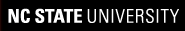# VH-1: A beginner's guide

VH-1 Home Introduction Quick start Inside the code
 Makefile indat vhone.f90 PPMLR Variable Glossary
Problems & solutions
 Riemann Sedov Rankine-Hugoniot Bondi DIY
This glossary lists only those variables that are used in vhone.f90 of the beginner's version, VH-0.5. Variables that are not declared in vhone.f90 are declared in PPMLR/vh1mods.f90. The 1D arrays listed here are declared a size (maxsweep), the value of which is nominally set to 1036 in PPMLR/vh1mods.f90. If you want more than 1024 zones in your grid, you will need to increase the value of maxsweep.

Variable name Variable type Description
dt REAL time step: time = time + dt for each cycle
dt3 REAL time step increased by 10%; new time step will not exceed this value
dtx REAL maximum allowable time step determined by CFL condition
dx0 REAL(:) array of zone widths; dx0 = dxfac
dxfac REAL width of every grid zone
e REAL(:) total energy (internal + kinetic); e(n) is the energy in zone n
endtime REAL maximum time to run program (not necessarily the ending time)
filename CHARACTER(50) output file for data at individual time steps
gam REAL ratio of specific heats
gamm REAL equal to (gam - 1.0), used to relate pressure to internal energy
hstfile CHARACTER(8) name of history file
i INTEGER index for x dimension of multi-D arrays
imax INTEGER number of physical zones in x dimension (not including ghost zones)
n INTEGER index for 1-D arrays
ncycle INTEGER current cycle number of time evolution
ncycend INTEGER maximum number of cycles to run program (not necessarily the ending cycle)
ncycp INTEGER number of cycles since last output file was written to disk
ndump INTEGER not used in VH-0.5
nfile INTEGER counter used to name output files; starts at zero and increments for each file written to disk
ngeom INTEGER flag used to specify geometry of the grid
nleft INTEGER flag used to specify boundary conditions for left edge of grid
nmax INTEGER index of last real zone on right side of grid, always = imax+6 (to account for 6 ghost zones)
nmin INTEGER index of first real zone on left side of grid, always = 7 (to account for 6 ghost zones)
nmovie INTEGER not used in VH-0.5
nprin INTEGER number of cycles executed between writing output files to disk
nright INTEGER flag used to specify boundary conditions for right edge of grid
p REAL(:) pressure; p(n) is the pressure in zone n
prefix CHARACTER(len=50) name used to prefix all output files
r REAL(:) density; r(n) is the density in zone n
ridt REAL maximum time to cross a zone at either fluid or sound speed; used to calculate CFL-constrained time step
rstrt CHARACTER(len=2) restart suffix: call init if = 'no', otherwise restart from dump file
svel REAL maximum time to cross a zone at sound speed; used to calculate CFL-constrained time step
sweep CHARACTER(len=1) label for current direction of hydrodynamic update; always = 'x' for 1D hydro in VH-0.5
time REAL current simulation time
timep REAL simulation time since last output file was written to disk
tmovie REAL not used in VH-0.5
todayis CHARACTER(len=8) Today's date as given by call to date_and_time; recorded in history file
tprin REAL simulation time between successive output files written to disk
u REAL(:) velocity; u(n) is the velocity in zone n
v REAL(:) transverse (y) velocity; typically set to zero in 1D hydro
w REAL(:) transverse (z) velocity; typically set to zero in 1D hydro
xa0 REAL(:) array of coordinates of the left edge of each zone on the grid
xmin REAL coordinate of left edge of the grid
xmax REAL coordinate of right edge of the grid
xvel REAL maximum time to cross a zone at the fluid speed; used to calculate CFL-constrained time step